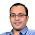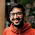### Post a Question

Have a question that you think you are not able to solve or even if you are able to solve it, you think fellow blog readers would like? Post as a comment on this page or add on the contact page. Thanks

1.please help me with this problem... find an infinite non constant arithmetic progression of positive integers such that each term is not a sum of two perfect cubes.

2.@Ayush,

Thanks for the question. Google search tells that it is USA IMO Team training problem. So, its not very simple :)

You can find the solution in the book: "104 number theory problems: from the training of the USA IMO team"

3.thanks a ton!!! i found the solution in the very book u mentioned.

4.please can u help me with this problem "in how many ways can u permute the digits 2,2,3,3,4,4,5,5 if the same digit must not appear in a row

5.Q1: Let f(n) denote the sum of the distinct prime divisors of the positive integer n and let d(n) denote the number of divisors of n. (So, for example, f(12) = 2 + 3 = 5 and d(12) = 6 since the divisors of 12 are 1,2, 3, 4, 6, and 12.)
Are there in finitely many n such that n*f(n) + d(n) is twice a perfect square?

Q2;A box contains 60 black and 40 white balls. Balls are randomly picked and removed from the box (without replacement) until the box is empty. As the balls are removed, the sequence of colors is recorded. A color change occurs when a ball is immediately followed by a ball of the other color. What is the expected number of color changes?

Q3:Four friends are reminiscing about a great party where they rst met many years ago. The fi rst friend can't remember the number of people at the party but does remember that it was a perfect square and must have been less than 5000. The second friend remembers the strange fact that each party guest had the same number of friends at the party. The third friend adds that no pair of friends shared a mutual friend at the party. And finally, the fourth recalls that every pair of non-friends had exactly 6 friends
in common amongst the party guests. How many people attended the party? (You should assume that
whenever person A is a friend of person B, person B is also a friend of person A.)

Q4:A single fair die is rolled fi ve times. The product of the rolls is then computed. Which result has a greater
probability of occurring: 180 or 144?

6.How many classes of subsets of the the set {1,2....n} follow this properties :
1) If set X and Y belong to class C then (X union Y) also belongs to C.
2) If set X and Y belong to class C then (X intersection Y) also belongs to C.

The problem roots from the open problem of counting topologies of any set with cardinality n.

7.Consider an array 'A' of 1st n natural numbers randomly permuted. Consider an array B formed from array A as given below
B(k) = number of elements from A(1) to A(k-1) which are smaller than A(k)
Obviously B(1) = 0;
i) Given A can you find B in O(n)
ii) Given B can you find A in O(n)

8.You are given a grid with m rows and n columns. Each cell is marked as either "b" or "w". You are also given black and white paint, and allowed k strokes of any paint. A stroke is defined as coloring of contiguous uncolored cells IN THE SAME ROW so the max length of a stroke is the no of columns, the moment you pick up your brush the stroke ends. The aim is to minimize the no of painting errors, an error occurs when you leave a cell unpainted or paint a "b" cell white or "w" cell black.

9.You are given a grid of m columns and n rows, each cell in this grid is marked either "b" or "w" indicating its supposed to painted Black or White. You are given black and white paints and k strokes of color of any of these colors. A stroke is defined as coloring of uncolored contiguous cells in the same row, the moment you pick up your brush, the stroke ends. The aim is to minimize the no of painting errors, an error occurs if a cell is colored in the wrong color or left uncolored. Come up with an optimal strategy.

10.@MASS: I am not able to solve the problem in first attempt. Looks like a graph theory problem to me. Can you please provide the source of the problem?

11.Two players compete in the following game: There is a pile containing n chips; the first player removes any number of chips except that he cannot take the whole pile. From then on, the players alternate moves, each person removing one or more chips but not more than twice as many chips as the preceding player has taken. The player who removes the last chip wins. (For example, suppose that n = 11; player A removes 3 chips; player B may remove up to 6 chips, and he takes 1. There remain 7 chips; player A may take 1 or 2 chips, and he takes 2;
player B may remove up to 4, and he picks up 1. There remain 4 chips; player A now takes 1; player B must take at least one chip and player A wins in the following

What is the best move for the first player to ensure his win if possible if there are initially n chips?

1.@akl.. please have a look at the discussion on
http://pratikpoddarcse.blogspot.in/2012/10/strategy-game-similar-to-nim.html

Thanks for the problem

12.Probability of choosing two points inside a square such that center of the square lies in the circle formed by taking the points as diameter

1.13.Q. Given a string of a, b, c, find the number of substrings with equal number of a, b and c. If the
input string is : ”abacbcba”, the output will be 3 (”acb”, ”cba”, ”acbcba”).
I can Do O(n^2) solution or may be better than that but i want a O(nlogn) solution .May be you can tell me ..please ..

1.Prepare an array of pairs where num[i] is (b_i,c_i) such that

b_i = (Number of b's from 0 to i) - (Number of a's from 0 to i)
c_i = (Number of c's from 0 to i) - (Number of a's from 0 to i)

You can get the array in O(n)

Now you need to find i and j such that the pair on i and jth indices are the same.
This can be done in O(n logn) - simple sorting.

Works for you? Thanks

14.I was asked this question in a job interview and I was not able to solve it .Given a complete graph with n vertices such that all edge wights are distinct .Prove that we can find a monotonically increasing path of length n-1 .

1.this is true provided vertex repetition is allowed in path , otherwise counter examples exist for n>=4.

suppose G is weighted graph. let s(v) denote length of longest monotonic path ending at v. let S denote summation of s(v) over all vertices v in G. start with a empty graph. clearly S=0. add the lowest weighted edge. S increases by 2......add the next higher weighted edge...and so on .....after n(n-1)/2 steps, the complete graph results.

in the aforesaid process value of S increases by at least 2 in each step after addition of an edge. the reason is as follows: if s(u) and s(v) were length of longest monotone path ending at vertices u and v respectively,then after addition of edge (u,v), we are assured a monotone path of length s(u)+1 ending at v and a monotone path of lentgh s(v)+1 ending at u.

so, after n(n-1)/2 steps, a complete graph results and S is at least n(n-1). Since S=sigma s(v), therefore there will exist a vertex v such that s(v)>=n-1.

hence proved.

15.Hi may i know how to substract the (ID)colum matrix from (K)square matrix as per equation below.

E = (K - ID)^-1 S

K is m*m matrix
I is idntity matrix
d is column vector
s is column vector
e is column vector

16.how many parts can an ellipse be divided into at maximum by n number of lines?please help me with this qsn

17.http://math.stackexchange.com/questions/555772/expected-number-of-points-on-circle-to-form-an-acute-angled-triangle/555889?noredirect=1#555889

18.This is an extension of a standard problem.
Source: Prof Sibi Raj Pillai, IIT Bombay ( for the UG course probability and random processes)

Say we have 100 people on a flight. The first person loses his ticket and sits at some arbitrary location. The other people sit at the seat allocated to them, or sit at some random location. In such a scenario, find the probability that the 100th person gets his own seat, given that the 2,5,10,12th people get their own seats while the 8th person doesn't get his own seat.

19.Hi Pratik,

The MIT Collective Intelligence Lab is crowdsourcing the next great IQ test. They are looking for people to create Raven's progressive matrices in order to develop an intelligence test that will be free to researchers. I thought you and your readers might want to know. They are offering \$2000 in prizes and those who submit will be listed as authors on paper about project. You can read story here: http://thepsychreport.com/current-events/news/mit-crowdsources-the-next-great-free-iq-test/

20.Given an array A of size n with elements from 1 to k and another Array B of size k with elements 1 to n. Show that they have a subarray of the same sum where n,k >= 1.

21.I found this cypher on 4chan and am hoping for some intelligent help...

https://i.4cdn.org/v/1521985220590.gif

(The postcard flips like ever 7 seconds so wait for the cypher.)
Thanks!!